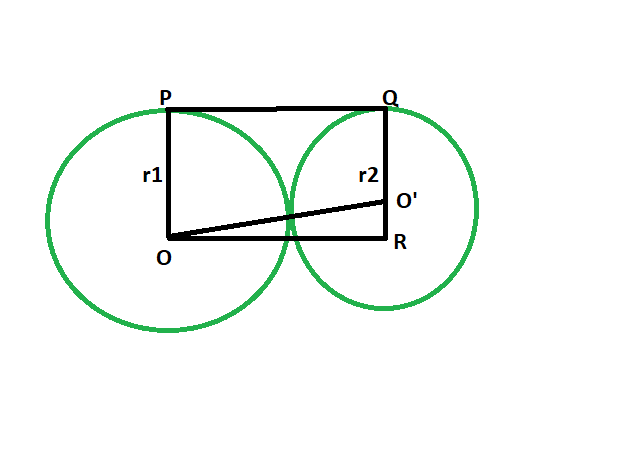GFG App
Open AppBrowser
Continue

# Length of the direct common tangent between two externally touching circles

Given two circles, of given radii, that touch each other externally. The task is to find the length of the direct common tangent between the circles.
Examples:

```Input: r1 = 5, r2 = 9
Output: 13.4164

Input: r1 = 11, r2 = 13
Output: 23.9165```Approach

• Let the radii be r1 & r2 respectively.
• Draw a line OR parallel to PQ
• angle OPQ = 90 deg
angle O’QP = 90 deg
{ line joining the centre of the circle to the point of contact makes an angle of 90 degrees with the tangent }
• angle OPQ + angle O’QP = 180
OP || QR
• Since opposite sides are parallel and interior angles are 90, therefore OPQR is a rectangle.
• So OP = QR = r1 and PQ = OR = r1+r2
• In triangle OO’R
angle ORO’ = 90
By Pythagoras theorem
OR^2 + O’R^2 = OO’^2
OO’^2 = (r1+r2)^2 + (r1-r2)^2
• So, OO’ = 2√(r1*r2)Below is the implementation of the above approach:

## C++

 `// C++ program to find the length of the direct` `// common tangent between two circles` `// which externally touch each other`   `#include ` `using` `namespace` `std;`   `// Function to find the length` `// of the direct common tangent` `void` `lengtang(``double` `r1, ``double` `r2)` `{` `    ``cout << ``"The length of the "` `         ``<< ``"direct common tangent is "` `         ``<< 2 * ``sqrt``(r1 * r2) << endl;` `}`   `// Driver code` `int` `main()` `{` `    ``double` `r1 = 5, r2 = 9;` `    ``lengtang(r1, r2);` `    ``return` `0;` `}`

## Java

 `// Java program to find the length of the direct ` `// common tangent between two circles ` `// which externally touch each other ` `class` `GFG ` `{`   `    ``// Function to find the length  ` `    ``// of the direct common tangent ` `    ``static` `void` `lengtang(``double` `r1, ``double` `r2) ` `    ``{` `        ``System.out.println(``"The length of the "` `                ``+ ``"direct common tangent is "` `                ``+ (``2` `* Math.sqrt(r1 * r2)));` `    ``}`   `    ``// Driver code ` `    ``public` `static` `void` `main(String[] args)` `    ``{` `        ``double` `r1 = ``5``, r2 = ``9``;` `        ``lengtang(r1, r2);` `    ``}` `}`   `// This code contributed by Rajput-Ji`

## Python3

 `# Python3 program to find the length ` `# of the direct common tangent ` `# between two circles which ` `# externally touch each other `   `# Function to find the length ` `# of the direct common tangent ` `def` `lengtang(r1, r2):` `    ``print``(``"The length of the direct"``, ` `                 ``"common tangent is"``, ` `             ``2` `*` `(r1 ``*` `r2)``*``*``(``1` `/` `2``)); `   `# Driver code ` `r1 ``=` `5``; r2 ``=` `9``; ` `lengtang(r1, r2); `   `# This code contributed ` `# by PrinciRaj1992 `

## C#

 `// C# program to find the length of the direct ` `// common tangent between two circles ` `// which externally touch each other` `using` `System;`   `class` `GFG` `{` `    ``// Function to find the length ` `    ``// of the direct common tangent ` `    ``static` `void` `lengtang(``double` `r1, ``double` `r2) ` `    ``{` `        ``Console.WriteLine(``"The length of the "` `                ``+ ``"direct common tangent is "` `                ``+ (2 * Math.Sqrt(r1 * r2)));` `    ``}` `    `  `    ``// Driver code ` `    ``static` `public` `void` `Main ()` `    ``{` `        ``double` `r1 = 5, r2 = 9;` `        ``lengtang(r1, r2);` `    ``}` `}`   `// This code contributed by ajit.`

## PHP

 ``

## Javascript

 ``

Output:

`The length of the direct common tangent is 13.4164`

Time Complexity: O(log(n)) because using inbuilt sqrt function

Auxiliary Space:  O(1)

My Personal Notes arrow_drop_up